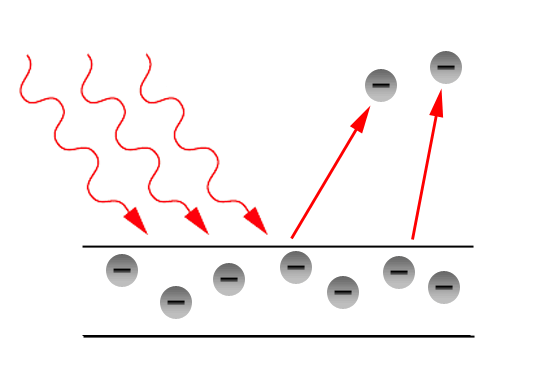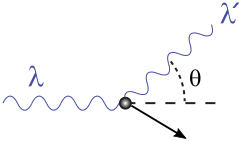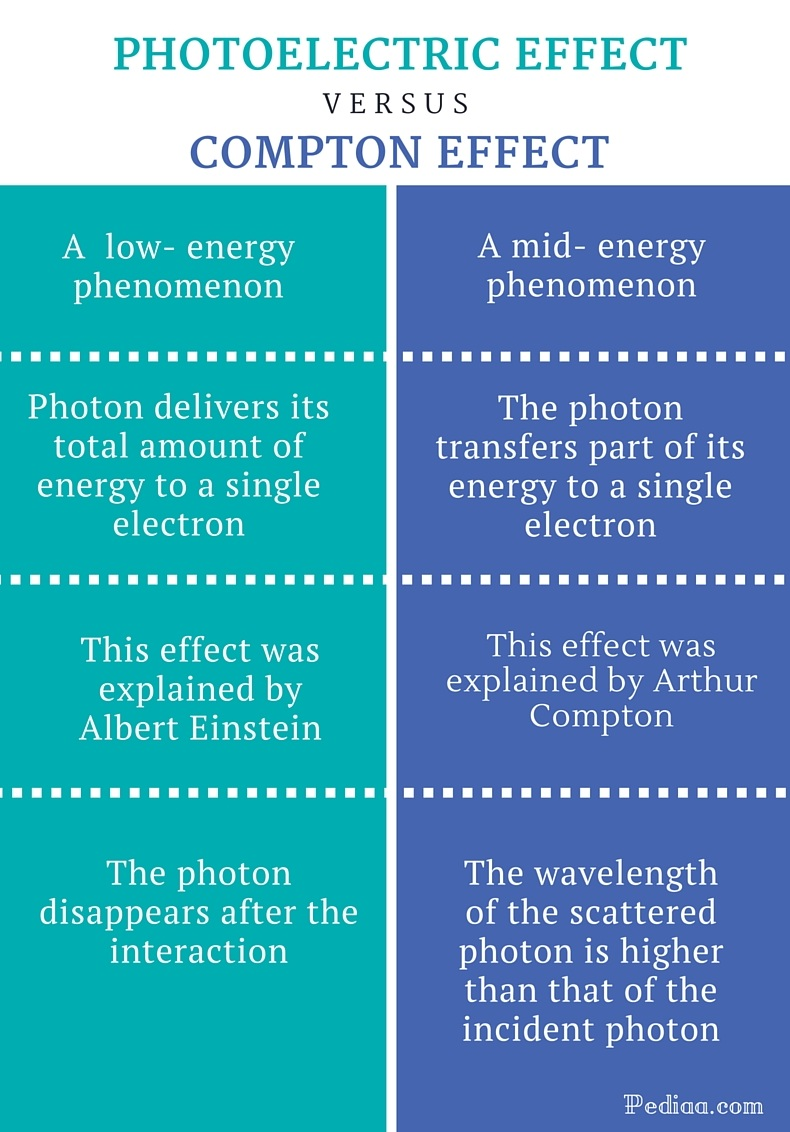# Difference Between Photoelectric Effect and Compton Effect

## Main Difference – Photoelectric Effect vs Compton Effect

The photoelectric effect and Compton effect are two types of interactions between light and matter. Both effects demonstrate the particle nature of electromagnetic waves. The photoelectric effect was explained by Albert Einstein. Compton effect was observed and explained by Arthur Compton. In the photoelectric effect, the entire energy of the incident photon is acquired by a single electron but, in the Compton effect, the incident photon transfers only a part of its energy to an electron. The photoelectric effect is a low energy phenomenon, and the interacted photons disappear just after they deliver their energy to electrons. The Compton effect, on the other hand, is a mid-energy phenomenon, and the interacted photons are scattered by the electrons. This is the main difference between Photoelectric Effect and Compton Effect.

## What is Photoelectric Effect

The photoelectric effect is an effect where weakly bound electrons within metals are ejected from the material when electromagnetic radiation interacts with those electrons. The ejected electrons are known as the photoelectrons. There are several important experimental observations relevant to the photoelectric effect. Some of them are;

1. The maximum kinetic energy of the photoelectrons (for a given material) depends only on the frequency of light.
2. The kinetic energies of photoelectrons are not dependent on the intensity of
3. There is a threshold frequency (cut-off frequency) which depends on the material. Light frequencies below the threshold frequency cannot produce photoelectrons.
4. The number of photoelectrons produced in the process is proportional to the intensity of light; higher the intensity, higher the number of photoelectrons.
5. Photoelectrons are ejected immediately after the material is illuminated.

The classical theory of electromagnetism cannot explain the above experimental observations (except the fourth observation). So, Albert Einstein developed a revolutionary theory to explain the photoelectric effect. He used the quantization idea of the electromagnetic radiation in his theory. According to his theory, light consists of energy packets or energy quanta called photons. They are absorbed or produced as units of energy packets. Simply, fractional energy packets do not exist. The energy (E) associated with a photon is given by; E= hf where, h = Planck’s constant and f =Frequency of the electromagnetic wave.

His theory suggests that the energy of a photon is completely acquired by a single electron in the metal. The electron spends a certain amount of energy (work function of the material) to liberate from its binding in the material. The electron comes out from the material as a liberated electron which is called a photoelectron. Normally, the electron loses some amount of its energy due to the interaction with other electrons in the surroundings on its way. The remaining energy of the electron appears as its kinetic energy. However, the energy is conserved in the process. So, the conservation of energy gives the relationship between the frequency of the incident photon and the kinetic energy of the photoelectron. It can be expressed as; hf = Ф + (K.E) where, Ф = work function of the material and K.E- Kinetic energy is of the photoelectron.

Einstein’s theory of photoelectric effect solved one of the challenging problems in physics. According to his theory, photoelectric effect demonstrates the particle nature of electromagnetic waves.## What is Compton Effect

Compton effect is the inelastic scattering of high-energy photons by loosely bound electrons or free charged particles. In this effect, the photon transfers part of its energy and momentum to the charged particle. So, the energy of the resultant photon is less than that of the incident photon. The wavelength of the scattered photon is higher than that of the incident photon as energy associated with a photon is inversely proportional to the wavelength of the photon. The charged particle that interacts with the photon acquires a part of energy and momentum of the photon and recoils. However, both the energy and momentum of the system is conserved in the process.

The Compton effect was observed by Arthur Compton, and the effect was named after his name. Compton developed a theoretical model to explain the Compton effect and eventually, he could derive a mathematical relationship between the change in the wavelength and the scattering angle of the photon. His equation can be expressed as, Δ λ = λ – λ0= h/mc (1 – cosθ)

where,

Δ λ- The change in the wavelength,

λ- Wavelength of the scattered photon,

λ0– Wavelength of the incident photon,

?- Scattering angle,

m- Mass of the electron,

h- Planck’s constant and,

The constant  is known as the Compton wavelength of the electron. It is equal to 2.43 10-12m. The scattering angle (00< ? < 1800) is the angle through which the photon is deflected. So, the wavelength shift becomes zero when the scattering angle is 00. On the other hand, the wavelength shift becomes twice the Compton wavelength of the electron (Maximum value of the wavelength shift) when the scattering angle is 1800.

The Compton effect is a good example of the particle nature of electromagnetic waves. The classical electromagnetic theory cannot explain the Compton effect or inelastic scattering of electromagnetic radiation. However, the classical theory can explain elastic scattering of electromagnetic radiation which is known as the Thomson scattering (Low- energy Compton scattering).

In the Compton effect, the fractional wavelength shift for low-energy photons (visible light, Infrared etc.) is very small. So, normally, Compton effect is important only for mid- energy photons such as X-ray or gamma ray photons.## Difference Between Photoelectric Effect and Compton Effect

### Phenomenon:

Photoelectric effect: Photoelectric effect is a low-energy phenomenon.

Compton effect: Compton effect is a mid-energy phenomenon.

### Energy:

Photoelectric effect: The photon delivers its total amount of energy to a single electron.

Compton effect: The photon transfers part of its energy to a single electron.

### First Theoretical Explanation:

Photoelectric effect: Photoelectric effect was explained by Albert Einstein.

Compton effect: Compton effect was explained by Arthur Compton.

### Fate of the Photon after the interaction:

Photoelectric effect: The photon disappears after the interaction.

Compton effect: The wavelength of the scattered photon is higher than that of the incident photon.Image Courtesy:

“Image 1”(CC BY-SA 3.0) via Commons Wikimedia

“Image 2” by JabberWok at the English language Wikipedia (CC BY-SA 3.0) via Commons Wikimedia